# Trace on a C*-algebra

(diff) ← Older revision | Latest revision (diff) | Newer revision → (diff)An additive functionalon the setof positive elements ofthat takes values in, is homogeneous with respect to multiplication by positive numbers and satisfies the condition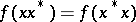for all. A traceis said to be finite iffor all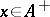, and semi-finite iffor all. The finite traces onare the restrictions toof those positive linear functionalsonsuch thatfor all. Letbe a trace on, letbe the set of elementssuch that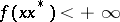, and let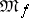be the set of linear combinations of products of pairs of elements of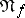. Thenandare self-adjoint two-sided ideals of, and there is a unique linear functionalonthat coincides withon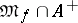. Letbe a lower semi-continuous semi-finite trace on a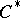-algebra. Then the formuladefines a Hermitian form on, with respect to which the mapping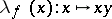ofinto itself is continuous for any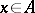. Put, and let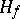be the completion of the quotient space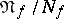with respect to the scalar product defined by the form. By passing to the quotient space and subsequent completion, the operatorsdetermine certain operatorson the Hilbert space, and the mapping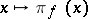is a representation of the-algebrain. The mappingestablishes a one-to-one correspondence between the set of lower semi-continuous semi-finite traces onand the set of representations ofwith a trace, defined up to quasi-equivalence.